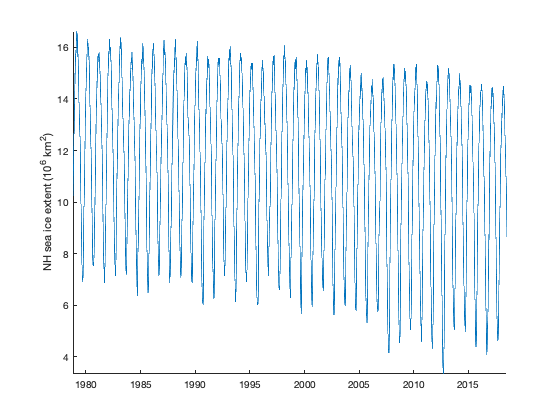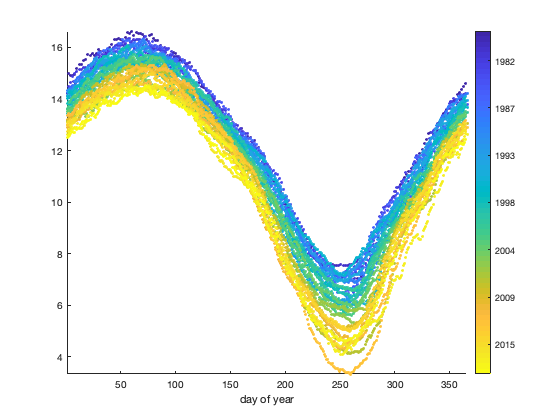# sinefit_bootstrap documentation

sinefit_bootstrap performs a bootstrap analysis on the parameters estimated by the function sinefit. Bootstrapping means applying the sinefit function to a bunch of subsamples of the data, then analyzing the distributions of solutions for each parameter to see how robust the solutions are.

For related functions, see sineval and sinefit documentation.

Back to Climate Data Tools Contents

## Syntax

```ft = sinefit_bootstrap(t,y)
ft = sinefit_bootstrap(...,'terms',TermOption)
ft = sinefit_bootstrap(...,'nboot',nboot)
[ft,rmse,Nsamp] = sinefit_bootstrap(...)```

## Description

ft = sinefit_bootstrap(t,y) fits a 2-term (amplitude and phase) sinusoid to 1000 random subsamples of the time series t,y. The output ft is a 1000x2 matrix containinng all 1000 solutions for the amplitude and phase, respectively. See sinefit for a complete description of inputs and outputs.

ft = sinefit_bootstrap(...,'terms',TermOption) specifies which terms are calculated in the sinusoid fit. Default is 2 because more terms can be computationally slow! TermOption can be 2, 3, 4, or 5:

• 2: ft = [A doy_max] where A is the amplitude of the sinusoid, and doy_max is the day of year corresponding to the maximum value of the sinusoid. The default TermOption is 2.
• 3: ft = [A doy_max C] also estimates C, a constant offset. Solving for adds processing time, so you may prefer to estimate C on your own simply as the mean of the input y. However, if you can't assume C=mean(y), you may prefer this three-term solution.
• 4: ft = [A doy_max C trend] also estimates a linear trend over the entire time series in units of y per year. Again, simultaneously solving for four terms will be much more computationally expensive than solving for two yerms, so you may prefer to estimate the trend on your own with polyfit, then calculate the two-term sine fit on your detrended data.
• 5: ft = [A doy_max C trend quadratic_term] also includes a quadratic term in the solution, but this is experimental for now, because fitting a polynomial to dates referenced to year zero tends to be scaled poorly.

ft = sinefit_bootstrap(...,'nboot',nboot) specifies the number of bootstrap samples. Default is 1000, meaning sinusoids are fit to 1000 random subsamples of the data.

[ft,rmse,Nsamp] = sinefit_bootstrap(...) also returns distributions of root-mean-square error of residuals (how well the sinusoids fit the data) and Nsamp, the number of datapoints contributing to each subsample of the data.

## Example

This example performs a bootstrap analysis of the phase and amplitude of a sea ice extent dataset. Load and plot the northern hemisphere sea ice extent time series:

```load seaice_extent

plot(t,extent_N)
box off
axis tight
ylabel 'NH sea ice extent (10^6 km^2)'
```Well that's about like interpreting a barcode. Try plotting as a function of day of the year for a better depiction of the seasonal cycle. I'm using my cbdate function for a date-formatted colorbar.

```figure
scatter(day(t,'dayofyear'),extent_N,10,datenum(t),'filled')
axis tight
box off
xlabel 'day of year'
cb = cbdate('yyyy');
set(cb,'ydir','reverse') % flips the direction of the colorbar
```Now the sinefit function can estimate the amplitude, phase, y-intercept, and slope of that time series all at once; however, computation gets quite a bit slower with every extra term, and sinefit_bootstrap will have to do the calculation a thousand times, so let's just detrend the data now for simplicity, and only solve for the phase and amplitude . The detrended time series looks like this:

```y = detrend(extent_N);

figure
plot(t,y)
box off
axis tight
ylabel 'detrended NH sea ice extent (10^6 km^2)'
```By eye we can see the amplitude of the sinusoid is about 4 million km^2, and this is the northern hemisphere sea ice extent, so we can guess that its maximum will be at the end of northern hemisphere winter or the spring. We can fit a 2-term sinusoid to the full time series to get something better than an eyeball estimation:

```sinefit(t,y)
```
```ans =
4.4085   66.8274
```

Those two numbers, 4.4 and 66.8, are the amplitude of the sinusoid and the day that the sinusoid reaches its maximum value. That is, 4.4 million km^2 and day 66 (March 7th). But how robust is that solution? Use sinefit_bootstrap to fit sinusoids to 1000 random samples of the sea ice extent time series (This solution will take about 20 seconds)

```ft = sinefit_bootstrap(t,y);

amp = ft(:,1);  % amplitude is the first column of ft
phase = ft(:,2);% phase is the second column of ft
```

If you have the Statistics Toolbox, you can use scatterhist to display the distributions of amplitude and phase solutions. Otherwise, you can just plot them in 2D as a scatterplot and/or plot the histograms of the individual distributions of the data:

```scatterhist(phase,amp)
xlabel 'day of sinusoid maximum'
ylabel 'amplitude (10^6 km^2)'
```Those are pretty tight clusters. They say that the amplitude and phase of the sinusoid fit should be accurate within about

```std(amp)
std(phase)
```
```ans =
0.0096
ans =
0.1028
```

0.009 km^2 and 0.1 days. That's not surprising, given that we have 40 years (40 cycles) of a very well sampled (365/cycle) dataset, we expect high accuracy of the sinefit amplitude and phase. But what if you only have a few data points, collected at random times? Can you still estimate a sinusoid? Here's what I mean: Let's trim down the dataset to just 7 random points:

```% Indices of 7 random data points:
ind = randi(length(y),[7 1]);

% Trim y and t to only those 7 points:
y = y(ind);
t = t(ind);

figure
plot(t,y,'bo')
axis tight
box off
ylabel 'NH sea ice extent (km^2)'
```That only looks like a few random points. But once again, plotting as a function of day of year can help us see what sinefit has to work with:

```figure
scatter(day(t,'dayofyear'),y,60,datenum(t),'filled')
axis tight
box off
xlabel 'day of year'
colorbar
cbdate('yyyy');
```So we see that sinefit has something like a sinusoid to approximate, even with only 7 points. It should not be surprising that with just these 7 points, sinefit still gets values pretty close to the well-constrained 4.4 amplitude with a maximum on day 66.8

```ft = sinefit(t,y)
```
```ft =
4.5210   72.4296
```

Here's what the sinusoid looks like on top of those 7 points:

```hold on
plot(1:365,sineval(ft,1:365))
```Use sinefit_bootstrap to quantify the uncertainty.

```ft = sinefit_bootstrap(t,y);

figure
scatterhist(ft(:,2),ft(:,1))
xlabel 'day of sinusoid maximum'
ylabel 'amplitude (km^2)'

amp_uncertainty = std(ft(:,1))
phase_uncertainty = std(ft(:,2))
```
```amp_uncertainty =
0.3966
phase_uncertainty =
4.7555
```And indeed, even with just 7 datapoints collected over 40 years, sinefit can estimate the amplitude within about 0.4 million km^2 and the phase within about 7 days.

## Note to users

One brief note related to the all the parameters estimated by sinefit: These parameters describe the best-fit sinusoid, but that does not necessarily mean they fully describe the behavior of the underlying data itself. For example, in terms of climatological average, Northern Hemisphere sea ice extent actually typically reaches a maximum around day 71 (March 12), whereas sinefit says the maximum value of the best-fit sinusoid occurs on day 66 (March 7). That's because the true behavior of sea ice extent is more complex than a simple sinusoid. In your work, be sure to consider the difference between true behavior and the 1/yr frequency component of the true behavior.

## Author Info

This function is part of the Climate Data Toolbox for Matlab. The sinefit, sineval, and sinefit_bootstrap functions as well as the supporting documentation were written by Chad A. Greene of the University of Texas Institute for Geophysics.